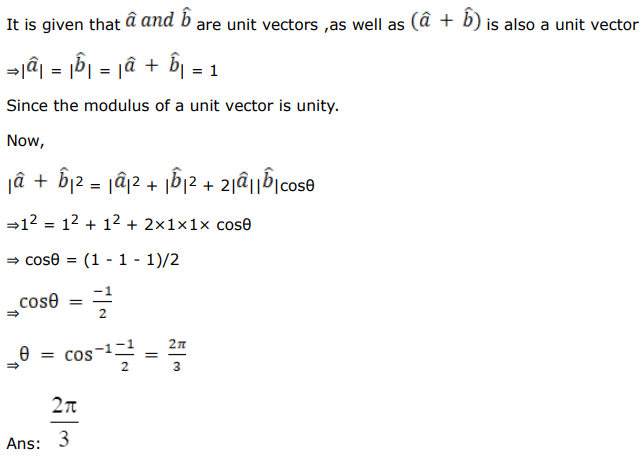# Solve this following

Question:

If $\hat{\mathrm{a}}$ and $\hat{\mathrm{b}}$ are unit vectors such that $(\hat{\mathrm{a}}+\mathrm{b})$ is a unit vector, what is the angle between $\hat{\mathrm{a}}$ and $\hat{\mathrm{b}}$ ?

Solution: